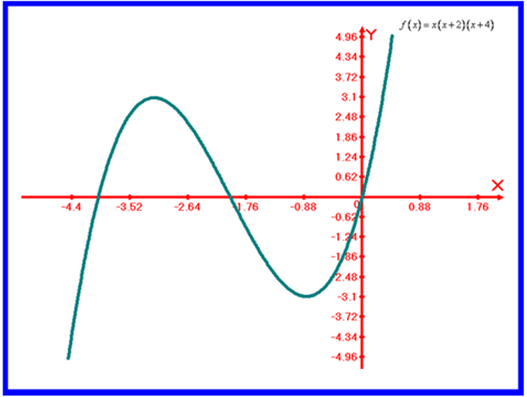# Use a graphical device; we sketch the graph of the function f ( x ) = | x ( x + 2 ) ( x + 4 ) |### Precalculus: Mathematics for Calcu...

6th Edition
Stewart + 5 others
Publisher: Cengage Learning
ISBN: 9780840068071### Precalculus: Mathematics for Calcu...

6th Edition
Stewart + 5 others
Publisher: Cengage Learning
ISBN: 9780840068071

#### Solutions

Chapter 2, Problem 50RE
To determine

## Use a graphical device; we sketch the graph of the function f(x)=|x(x+2)(x+4)|

Expert Solution

Sketch the graph of the function f(x)=|x(x+2)(x+4)| as shown below:### Explanation of Solution

Given information: consider the function f(x)=|x(x+2)(x+4)|

Calculation:

Use a graphical device; we sketch the graph of the function f(x)=|x(x+2)(x+4)| as shown below:### Have a homework question?

Subscribe to bartleby learn! Ask subject matter experts 30 homework questions each month. Plus, you’ll have access to millions of step-by-step textbook answers!